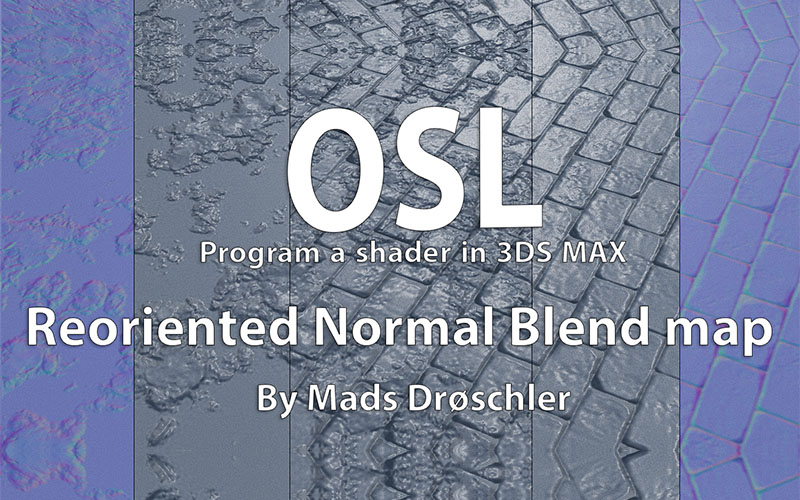## OSL | 混合法线贴图

603 1 2021-05-10

``````// NormalBlend.osl
// Last update 10.12.2019
// Based on an article by Colin Barré-Brisebois and Stephen Hill regarding different normal blend types.

vector rnm(vector A, vector B) {
vector a = A * vector( 2,  2, 2) + vector(-1, -1,  0);
vector b = B * vector(-2, -2, 2) + vector( 1,  1, -1);
vector r = a * dot(a, b) / a - b;
return r * 0.5 + 0.5;
}

[[
string help = "Use this map to blend normals, the blend method is reoriented and superior to many other blend types. Remember to change your input to linear space, where gamma = 1.0",

]]
(
color NormalA = color(0.5,0.5,1.0),
int Enable_NormalA = 1
[[
string widget = "checkBox",
]],
float MixA = 1.0
[[
float min = 0,
float max = 1
]],
color NormalB = color(0.5,0.5,1.0),
int Enable_NormalB = 1
[[
string widget = "checkBox",
]],
float MixB = 1.0
[[
float min = 0,
float max = 1
]],
int FlipOrder = 0
[[
string widget = "checkBox",
]],

output color Out = 0,
)
{
color C = color(0.5,0.5,1.0);
color pipeA,pipeB = 0;

if ( FlipOrder == 0 )
{
pipeA = NormalA;
pipeB = NormalB;
}
else
{
pipeA = NormalB;
pipeB = NormalA;
}

if ( Enable_NormalA == 1 )
{
pipeA = mix(pipeA,C,1-MixA);
}
else
{
pipeA = C;
}
if( Enable_NormalB == 1 )
{
pipeB = mix(pipeB,C,1-MixB);
}
else
{
pipeB = C;
}

Out = rnm(pipeA,pipeB);
}``````